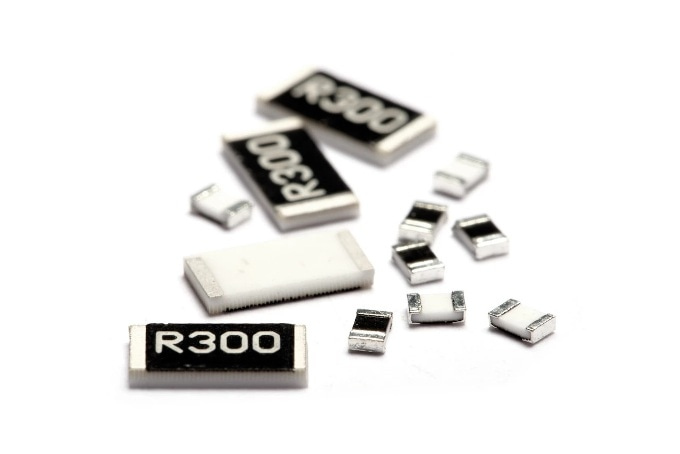# An Introduction to Cost-Effective Current Flow MeasurementThe relative relationship between voltage and current, according to Ohm’s Law, allows a sensor circuit to measure the amperes distributed to a load in real-time.  This is a simple and cost-effective way of measuring current flow and alleviates the effects of short-circuits or overloads. It also maximizes the overall efficiency of electrical systems. Nevertheless, there are certain considerations to be mindful of when measuring the flow of a current with a current sense resistor.

In this article, TT Electronics covers the foundations of current sense resistors in more detail.

## Current Sense Resistors: The Basics

Typically, current sense resistors are placed in-line on the conduction path between the power source and the load in an IC or PCB. The part is usually engineered for extremely low resistance values in order to abate the voltage drop and the power loss. Normal resistance values for current sense resistors fall on the milliohms scale.

The overarching theory of current sense resistors follows the working principles of standard electrical resistors. However, a standard resistor is typically used to regulate the current. In contrast, a current sense resistor is used for monitoring purposes.

Current sense resistors are frequently coupled with amplifiers to reduce noise impact while upholding precise resolution levels. Where the ensuing circuitry runs at a different voltage from the current path, an isolation amplifier is used. While this is not a compulsory configuration, choosing between isolated and non-isolated current sense resistors is a central question to consider when designing a system for current flow measurement.

When choosing a current sense resistor, considering the voltage drop metric is key. Interposing the current sense resistor between the supply and the load will result in a degree of power dissipation due to the voltage drop occurring as a result of the current passing through the resistor. This constitutes wasted power that is dissipated as heat instead of reaching the load. This can further impact the performance of the device.

## Current Sense Resistors from TT Electronics

TT Electronics is one of the world’s top suppliers of precision electrical components. We have offered cost-effective current sense resistors, all RoHS compliant, for a huge range of applications, equipping engineers with effective solutions for current flow measurement in an array electronics sectors.This information has been sourced, reviewed and adapted from materials provided by TT Electronics plc.

## Citations

• APA

TT Electronics plc. (2019, October 29). An Introduction to Cost-Effective Current Flow Measurement. AZoSensors. Retrieved on September 19, 2020 from https://www.azosensors.com/article.aspx?ArticleID=1754.

• MLA

TT Electronics plc. "An Introduction to Cost-Effective Current Flow Measurement". AZoSensors. 19 September 2020. <https://www.azosensors.com/article.aspx?ArticleID=1754>.

• Chicago

TT Electronics plc. "An Introduction to Cost-Effective Current Flow Measurement". AZoSensors. https://www.azosensors.com/article.aspx?ArticleID=1754. (accessed September 19, 2020).

• Harvard

TT Electronics plc. 2019. An Introduction to Cost-Effective Current Flow Measurement. AZoSensors, viewed 19 September 2020, https://www.azosensors.com/article.aspx?ArticleID=1754.

## Tell Us What You Think